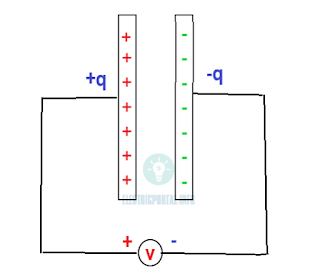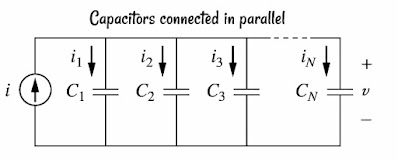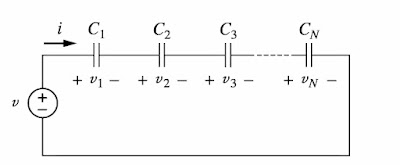# Working Of Capacitor, Formula, Symbol Properties

Capacitor is a passive element in a circuit designed to store energy in the form of an electric field. besides resistor, capacitors are the most common electrical components. used mostly in electronics circuit, computers, power system. They are also used in tunning circuits of radio receivers.

## Working of Capacitor

A capacitor consists of two conducting plates separated by a dielectric medium like mica, ceramic, paper or air.

Symbol of the fixed and variable capacitor is shown in the below diagramFixed and variable capacitor symbol

When a voltage source say "V" is connected across the two plates of the capacitor as shown in the diagram. The source deposits a positive charge "+q" on one plate and a negative charge on the other side plate (-q). It stores energy in the form of electric charge. the amount of charge stored represent by "q" is directly proportional to the applied voltage V.Capacitor Diagram

Hence,
q=cv
Where "c" is constant of proportionality called as the capacitance of the capacitor and the unit is farad represented by F.

Capacitance is defined as the ratio of the charge on one plate of a capacitor to the voltage difference between the two plates.

Capacitors are used to block DC current and allow AC current, shift phase, store energy, to start the motor (single-phase induction motor) and to suppress noise, these are the main functions.

Voltage current relation formula,

i=dq/dt
i=c*dv/dt

### Capacitors connected in parallelparallel connected capacitors

Total equivalent capacitance calculation formula for parallel-connected capacitors

Ceq =C₁+C₂+C₃+...........Cₙ

Equivalent capacitance of parallel connect capacitors is the sum of individual capacitance.

### Capacitors connected in seriesSeries connected capacitors

Total equivalent capacitance calculation formula for series-connected capacitors

1/Ceq=1/C₁+1/C₂+1/C₃+..........1/Cₙ

Equivalent capacitance of series-connected Capacitors is reciprocal of the sum of the reciprocal of the individual capacitance.

### Important properties of the capacitor

• When the voltage across the capacitor is not changing with time( Dc voltage) current through the capacitor is zero and the capacitor acts as an open circuit for DC current.
• The voltage on the capacitor cannot changed suddenly.
• Capacitor resists sudden change in voltage across it.